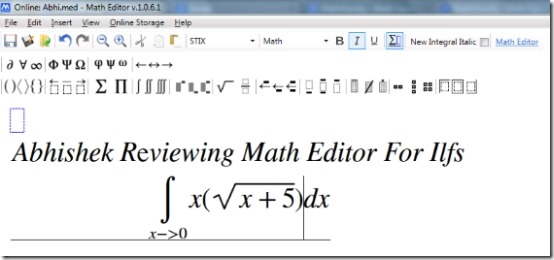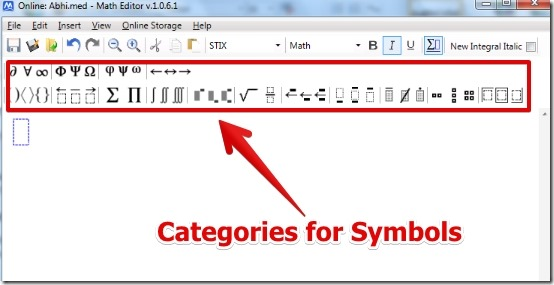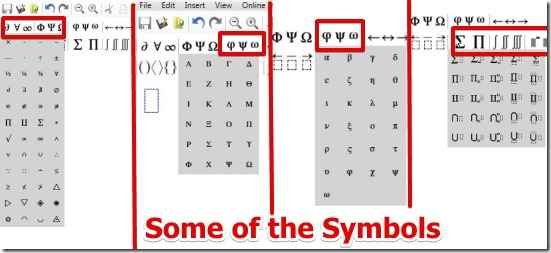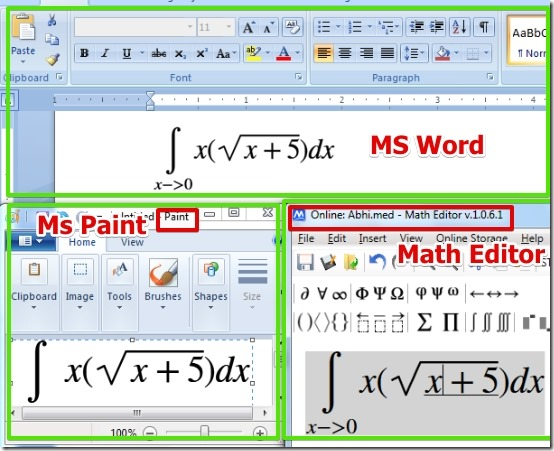Editor Ratings:
User Ratings:
[Total: 4 Average: 4.3]

Math Editor is free software using which you can write Math equation with numerous symbols. The symbols include integrals, partial intergrals, square root,  Greek symbols (alpha, beta etc.), Cyrillic, and many more. Math Editor lets you save the work in .png, .jpeg, .bmp, .gif, .tiff, and .wmpp formats. Also, Math Editor lets you copy the Math equation and paste it into MS word and in MS Paint as an image.

I have run Math Editor in Windows 7 and I was able to write good Math equation very easily. Actually, Math Editor worked really good for me.## User Interface of Math Equation Editor:

When you start the Math Editor you see the simple and very easy to understand user interface.Math Editor has well defined symbol categories on the top from where you can select the proper symbol buttons very easily. However, you can search for symbols under the Insert – > Symbol option also according to the names.

## How To Use Math Equation Editor:

Using Math Editor is really easy. You have to just click on the symbol category from the Menu and simply select the symbol and place it on the space provided for the equation. After that you can input the values by using the keyboard. Below I have shown some of the symbols but you get a lot more symbols than these.Now if you are working in Word or Paint and you want to insert a Math equation then you would probably draw it in paint or find the font for the same. But using Math Editor you can simply create the equation in it and paste it in Word or MS Paint as an image. This method requires way less effort and saves a lot of time.What I really liked about this Equation Editor along with the symbols is that, you can even use the keyboard for the typing of the equation. It is really good as you are not bounded to use only unknown x and y as variables for the equation. You can write any variable name it whatever you like. Actually you can write anything as you write in Word.

One more thing that I liked in Math Editor is that you can copy the equation or any text and simply repeat the equation without having to type whole equation all over again. You can access this under the Edit Menu for copying the Math equation text or you can use shortcut for that which is ctrl + c for copy and ctrl + v for paste.

After your work is done, you can save it or you can export as an image file to popular image formats such as .jpg, .png, .gif, and many more. Also, you can save the work in your online account directly from Math Editor and retrieve it anytime by logging into your account from Math Editor Software.

Note: Math Editor requires .Net Framework to run. Your computer would most probably already have it installed.

Also, you need to have an account before you can download the Math Editor. Registration is completely free.

## Features of Equation Editor:

• Very easy to use.
• Large number of symbols for writing the Math equation.
• Option to export the file in .jpg, . png, and many more formats.
• Cut, copy, and paste option for the equation.
• Option to use the Math equation in MS Word and MS paint as image.
• Option to save the work online into your account and view it anywhere using the Math Editor Software.

You can also try Lurch. It is free computer aided instruction software using which you can check how good is the Math problem in your text documents are.

## Conclusion:

Math Editor is great software when you want to write a Math Equation in your computer. Also, the equation you write can be used in MS Word and MS Paint as an image. Based on the simple interface, large support for symbols, and great features I would recommend you to try it out.